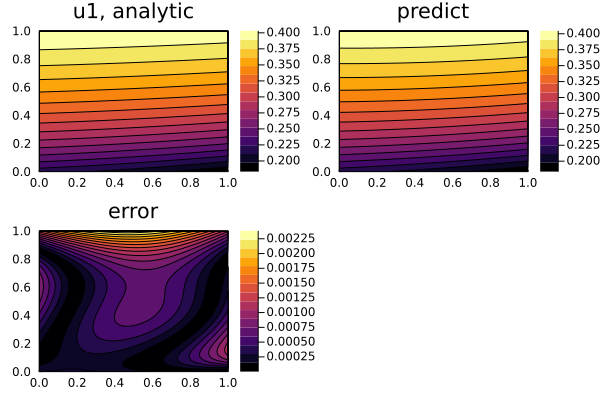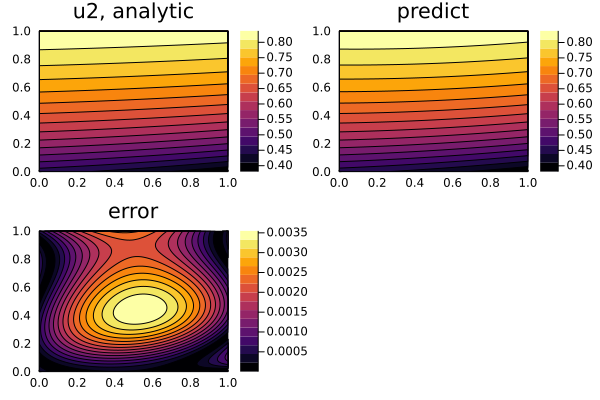# Systems of PDEs

In this example, we will solve the PDE system:

\begin{align*} ∂_t u_1(t, x) & = ∂_x^2 u_1(t, x) + u_3(t, x) \, \sin(\pi x) \, ,\\ ∂_t u_2(t, x) & = ∂_x^2 u_2(t, x) + u_3(t, x) \, \cos(\pi x) \, ,\\ 0 & = u_1(t, x) \sin(\pi x) + u_2(t, x) \cos(\pi x) - e^{-t} \, , \end{align*}

with the initial conditions:

\begin{align*} u_1(0, x) & = \sin(\pi x) \, ,\\ ∂_t u_1(0, x) & = - \sin(\pi x) \, ,\\ u_2(0, x) & = \cos(\pi x) \, ,\\ ∂_t u_2(0, x) & = - \cos(\pi x) \, , \end{align*}

and the boundary conditions:

\begin{align*} u_1(t, 0) & = u_1(t, 1) = 0 \, ,\\ u_2(t, 0) & = - u_2(t, 1) = e^{-t} \, , \end{align*}

with physics-informed neural networks.

using NeuralPDE, Flux, ModelingToolkit, GalacticOptim, Optim, DiffEqFlux
import ModelingToolkit: Interval, infimum, supremum

@parameters t, x
@variables u1(..), u2(..), u3(..)
Dt = Differential(t)
Dtt = Differential(t)^2
Dx = Differential(x)
Dxx = Differential(x)^2

eqs = [Dtt(u1(t,x)) ~ Dxx(u1(t,x)) + u3(t,x)*sin(pi*x),
Dtt(u2(t,x)) ~ Dxx(u2(t,x)) + u3(t,x)*cos(pi*x),
0. ~ u1(t,x)*sin(pi*x) + u2(t,x)*cos(pi*x) - exp(-t)]

bcs = [u1(0,x) ~ sin(pi*x),
u2(0,x) ~ cos(pi*x),
Dt(u1(0,x)) ~ -sin(pi*x),
Dt(u2(0,x)) ~ -cos(pi*x),
u1(t,0) ~ 0.,
u2(t,0) ~ exp(-t),
u1(t,1) ~ 0.,
u2(t,1) ~ -exp(-t)]

# Space and time domains
domains = [t ∈ Interval(0.0,1.0),
x ∈ Interval(0.0,1.0)]

# Neural network
input_ = length(domains)
n = 15
chain =[FastChain(FastDense(input_,n,Flux.σ),FastDense(n,n,Flux.σ),FastDense(n,1)) for _ in 1:3]
initθ = map(c -> Float64.(c), DiffEqFlux.initial_params.(chain))

discretization = PhysicsInformedNN(chain, _strategy, init_params= initθ)

@named pde_system = PDESystem(eqs,bcs,domains,[t,x],[u1(t, x),u2(t, x),u3(t, x)])
prob = discretize(pde_system,discretization)
sym_prob = symbolic_discretize(pde_system,discretization)

pde_inner_loss_functions = prob.f.f.loss_function.pde_loss_function.pde_loss_functions.contents
bcs_inner_loss_functions = prob.f.f.loss_function.bcs_loss_function.bc_loss_functions.contents

cb = function (p,l)
println("loss: ", l )
println("pde_losses: ", map(l_ -> l_(p), pde_inner_loss_functions))
println("bcs_losses: ", map(l_ -> l_(p), bcs_inner_loss_functions))
return false
end

res = GalacticOptim.solve(prob,BFGS(); cb = cb, maxiters=5000)

phi = discretization.phi

Low-level api

using NeuralPDE, Flux, ModelingToolkit, GalacticOptim, Optim, DiffEqFlux
import ModelingToolkit: Interval, infimum, supremum

@parameters t, x
@variables u1(..), u2(..), u3(..)
Dt = Differential(t)
Dtt = Differential(t)^2
Dx = Differential(x)
Dxx = Differential(x)^2

eqs = [Dtt(u1(t,x)) ~ Dxx(u1(t,x)) + u3(t,x)*sin(pi*x),
Dtt(u2(t,x)) ~ Dxx(u2(t,x)) + u3(t,x)*cos(pi*x),
0. ~ u1(t,x)*sin(pi*x) + u2(t,x)*cos(pi*x) - exp(-t)]

bcs = [u1(0,x) ~ sin(pi*x),
u2(0,x) ~ cos(pi*x),
Dt(u1(0,x)) ~ -sin(pi*x),
Dt(u2(0,x)) ~ -cos(pi*x),
u1(t,0) ~ 0.,
u2(t,0) ~ exp(-t),
u1(t,1) ~ 0.,
u2(t,1) ~ -exp(-t)]

# Space and time domains
domains = [t ∈ Interval(0.0,1.0),
x ∈ Interval(0.0,1.0)]

# Neural network
input_ = length(domains)
n = 15
chain =[FastChain(FastDense(input_,n,Flux.σ),FastDense(n,n,Flux.σ),FastDense(n,1)) for _ in 1:3]
initθ = map(c -> Float64.(c), DiffEqFlux.initial_params.(chain))
flat_initθ = reduce(vcat,initθ )

eltypeθ = eltype(initθ)
parameterless_type_θ = DiffEqBase.parameterless_type(initθ)
phi = NeuralPDE.get_phi.(chain,parameterless_type_θ)

map(phi_ -> phi_(rand(2,10), flat_initθ),phi)

derivative = NeuralPDE.get_numeric_derivative()

indvars = [t,x]
depvars = [u1,u2,u3]
dim = length(domains)

_pde_loss_functions = [NeuralPDE.build_loss_function(eq,indvars,depvars,phi,derivative,

map(loss_f -> loss_f(rand(2,10), flat_initθ),_pde_loss_functions)

bc_indvars = NeuralPDE.get_argument(bcs,indvars,depvars)
_bc_loss_functions = [NeuralPDE.build_loss_function(bc,indvars,depvars, phi, derivative,
bc_indvars = bc_indvar) for (bc,bc_indvar) in zip(bcs,bc_indvars)]
map(loss_f -> loss_f(rand(1,10), flat_initθ),_bc_loss_functions)

# dx = 0.1
# train_sets = NeuralPDE.generate_training_sets(domains,dx,eqs,bcs,eltypeθ,indvars,depvars)
# pde_train_set,bcs_train_set = train_sets

plbs,pubs = pde_bounds
pde_loss_functions = [NeuralPDE.get_loss_function(_loss,
lb,ub,
eltypeθ, parameterless_type_θ,
for (_loss,lb,ub) in zip(_pde_loss_functions, plbs,pubs)]

map(l->l(flat_initθ) ,pde_loss_functions)

blbs,bubs = bcs_bounds
bc_loss_functions = [NeuralPDE.get_loss_function(_loss,lb,ub,
eltypeθ, parameterless_type_θ,
for (_loss,lb,ub) in zip(_bc_loss_functions, blbs,bubs)]

map(l->l(flat_initθ) ,bc_loss_functions)

loss_functions =  [pde_loss_functions;bc_loss_functions]

function loss_function(θ,p)
sum(map(l->l(θ) ,loss_functions))
end

f_ = OptimizationFunction(loss_function, GalacticOptim.AutoZygote())
prob = GalacticOptim.OptimizationProblem(f_, flat_initθ)

cb_ = function (p,l)
println("loss: ", l )
println("pde losses: ", map(l -> l(p), loss_functions[1:3]))
println("bcs losses: ", map(l -> l(p), loss_functions[4:end]))
return false
end

res = GalacticOptim.solve(prob,Optim.BFGS(); cb = cb_, maxiters=5000)

And some analysis for both low and high level api:

using Plots

ts,xs = [infimum(d.domain):0.01:supremum(d.domain) for d in domains]

acum =  [0;accumulate(+, length.(initθ))]
sep = [acum[i]+1 : acum[i+1] for i in 1:length(acum)-1]
minimizers_ = [res.minimizer[s] for s in sep]

analytic_sol_func(t,x) = [exp(-t)*sin(pi*x), exp(-t)*cos(pi*x), (1+pi^2)*exp(-t)]
u_real  = [[analytic_sol_func(t,x)[i] for t in ts for x in xs] for i in 1:3]
u_predict  = [[phi[i]([t,x],minimizers_[i]) for t in ts  for x in xs] for i in 1:3]
diff_u = [abs.(u_real[i] .- u_predict[i] ) for i in 1:3]
for i in 1:3
p1 = plot(ts, xs, u_real[i],linetype=:contourf,title = "u$i, analytic"); p2 = plot(ts, xs, u_predict[i],linetype=:contourf,title = "predict"); p3 = plot(ts, xs, diff_u[i],linetype=:contourf,title = "error"); plot(p1,p2,p3) savefig("sol_u$i")
end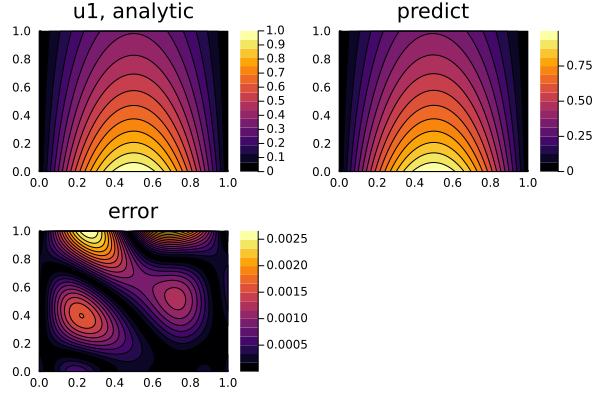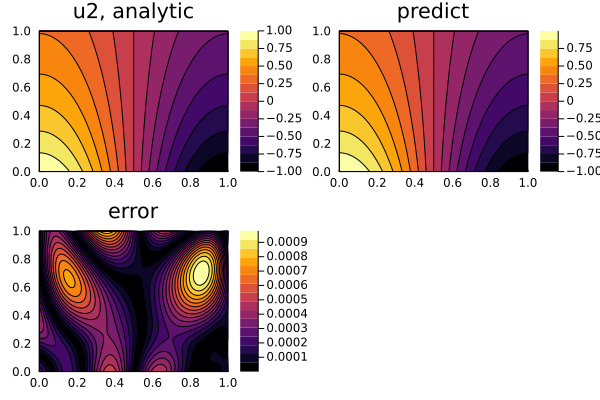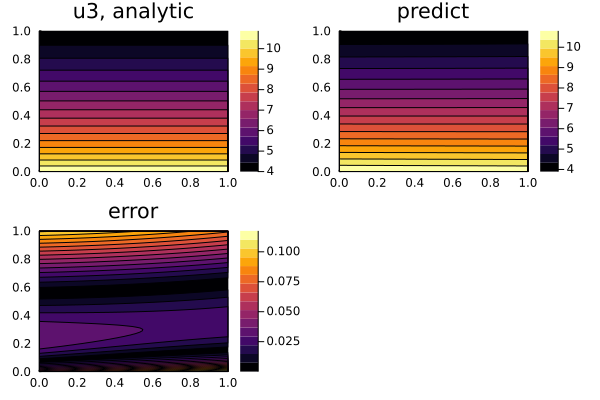## Derivative neural network approximation

The accuracy and stability of numerical derivative decreases with each successive order. The accuracy of the entire solution is determined by the worst accuracy of one of the variables, in our case - the highest degree of the derivative. Derivative neural network approximation is such an approach that using lower-order numeric derivatives and estimates higher-order derivatives with a neural network so that allows an increase in the marginal precision for all optimization.

Since u3 is only in the first and second equations, that its accuracy during training is determined by the accuracy of the second numerical derivative u3(t,x) ~ (Dtt(u1(t,x)) -Dxx(u1(t,x))) / sin(pi*x).

We approximate the derivative of the neural network with another neural network Dt(u1(t,x)) ~ Dtu1(t,x) and train it along with other equations, and thus we avoid using the second numeric derivative Dt(Dtu1(t,x)).

using NeuralPDE, Flux, ModelingToolkit, GalacticOptim, Optim, DiffEqFlux
import ModelingToolkit: Interval, infimum, supremum

@parameters t, x
Dt = Differential(t)
Dx = Differential(x)
@variables u1(..), u2(..), u3(..)
@variables Dxu1(..),Dtu1(..),Dxu2(..),Dtu2(..)

eqs_ = [Dt(Dtu1(t,x)) ~ Dx(Dxu1(t,x)) + u3(t,x)*sin(pi*x),
Dt(Dtu2(t,x)) ~ Dx(Dxu2(t,x)) + u3(t,x)*cos(pi*x),
exp(-t) ~ u1(t,x)*sin(pi*x) + u2(t,x)*cos(pi*x)]

bcs_ = [u1(0.,x) ~ sin(pi*x),
u2(0.,x) ~ cos(pi*x),
Dt(u1(0,x)) ~ -sin(pi*x),
Dt(u2(0,x)) ~ -cos(pi*x),
#Dtu1(0,x) ~ -sin(pi*x),
# Dtu2(0,x) ~ -cos(pi*x),
u1(t,0.) ~ 0.,
u2(t,0.) ~ exp(-t),
u1(t,1.) ~ 0.,
u2(t,1.) ~ -exp(-t)]

der_ = [Dt(u1(t,x)) ~ Dtu1(t,x),
Dt(u2(t,x)) ~ Dtu2(t,x),
Dx(u1(t,x)) ~ Dxu1(t,x),
Dx(u2(t,x)) ~ Dxu2(t,x)]

bcs__ = [bcs_;der_]

input_ = length(domains)
n = 15
chain = [FastChain(FastDense(input_,n,Flux.σ),FastDense(n,n,Flux.σ),FastDense(n,1)) for _ in 1:7]
initθ = map(c -> Float64.(c), DiffEqFlux.initial_params.(chain))

grid_strategy = NeuralPDE.GridTraining(0.07)
discretization = NeuralPDE.PhysicsInformedNN(chain,
grid_strategy,
init_params= initθ)

vars = [u1(t,x),u2(t,x),u3(t,x),Dxu1(t,x),Dtu1(t,x),Dxu2(t,x),Dtu2(t,x)]
@named pde_system = PDESystem(eqs_,bcs__,domains,[t,x],vars)
prob = NeuralPDE.discretize(pde_system,discretization)
sym_prob = NeuralPDE.symbolic_discretize(pde_system,discretization)

pde_inner_loss_functions = prob.f.f.loss_function.pde_loss_function.pde_loss_functions.contents
inner_loss_functions = prob.f.f.loss_function.bcs_loss_function.bc_loss_functions.contents
bcs_inner_loss_functions = inner_loss_functions[1:7]
aprox_derivative_loss_functions = inner_loss_functions[9:end]

cb = function (p,l)
println("loss: ", l )
println("pde_losses: ", map(l_ -> l_(p), pde_inner_loss_functions))
println("bcs_losses: ", map(l_ -> l_(p), bcs_inner_loss_functions))
println("der_losses: ", map(l_ -> l_(p), aprox_derivative_loss_functions))
return false
end

res = GalacticOptim.solve(prob, ADAM(0.01); cb = cb, maxiters=2000)
prob = remake(prob,u0=res.minimizer)
res = GalacticOptim.solve(prob,BFGS(); cb = cb, maxiters=10000)

phi = discretization.phi

And some analysis:

using Plots

ts,xs = [infimum(d.domain):0.01:supremum(d.domain) for d in domains]

initθ = discretization.init_params
acum =  [0;accumulate(+, length.(initθ))]
sep = [acum[i]+1 : acum[i+1] for i in 1:length(acum)-1]
minimizers_ = [res.minimizer[s] for s in sep]

u1_real(t,x) = exp(-t)*sin(pi*x)
u2_real(t,x) = exp(-t)*cos(pi*x)
u3_real(t,x) = (1+pi^2)*exp(-t)
Dxu1_real(t,x) = pi*exp(-t)*cos(pi*x)
Dtu1_real(t,x) = -exp(-t)*sin(pi*x)
Dxu2_real(t,x) = -pi*exp(-t)*sin(pi*x)
Dtu2_real(t,x) = -exp(-t)*cos(pi*x)
analytic_sol_func_all(t,x) = [u1_real(t,x), u2_real(t,x), u3_real(t,x),
Dxu1_real(t,x),Dtu1_real(t,x),Dxu2_real(t,x),Dtu2_real(t,x)]

u_real  = [[analytic_sol_func_all(t,x)[i] for t in ts for x in xs] for i in 1:7]
u_predict  = [[phi[i]([t,x],minimizers_[i]) for t in ts  for x in xs] for i in 1:7]
diff_u = [abs.(u_real[i] .- u_predict[i] ) for i in 1:7]

titles = ["u1","u2","u3","Dtu1","Dtu2","Dxu1","Dxu2"]
for i in 1:7
p1 = plot(ts, xs, u_real[i], linetype=:contourf,title = "$(titles[i]), analytic"); p2 = plot(ts, xs, u_predict[i], linetype=:contourf,title = "predict"); p3 = plot(ts, xs, diff_u[i],linetype=:contourf,title = "error"); plot(p1,p2,p3) savefig("3sol_ub$i")
end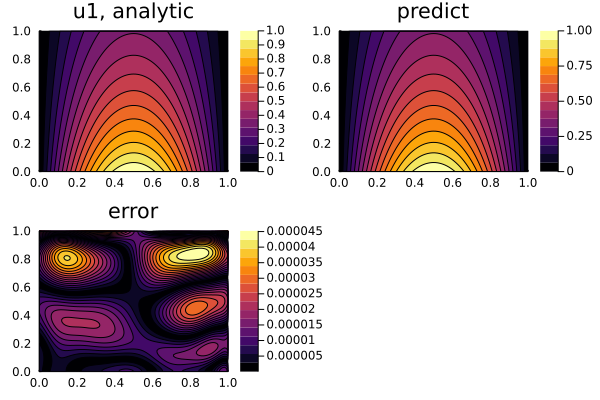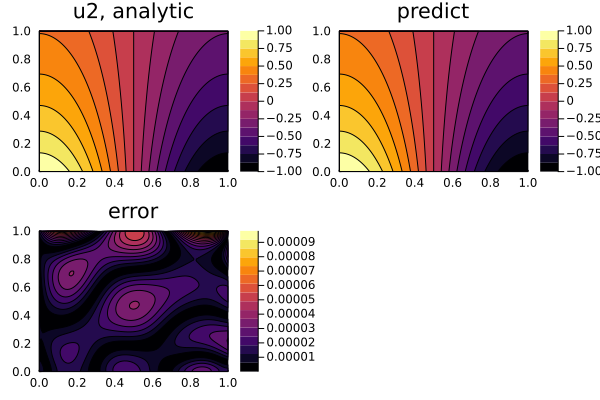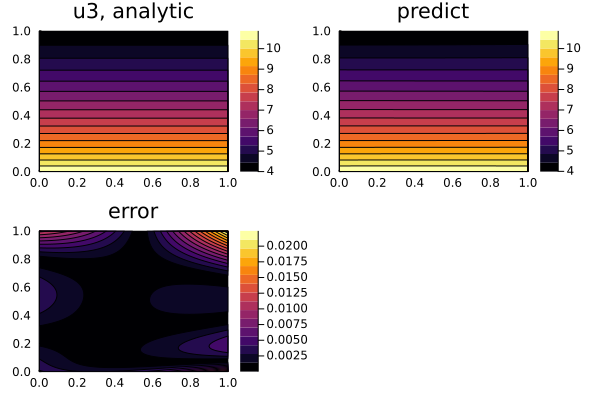## Comparison of the second numerical derivative and numerical + neural network derivative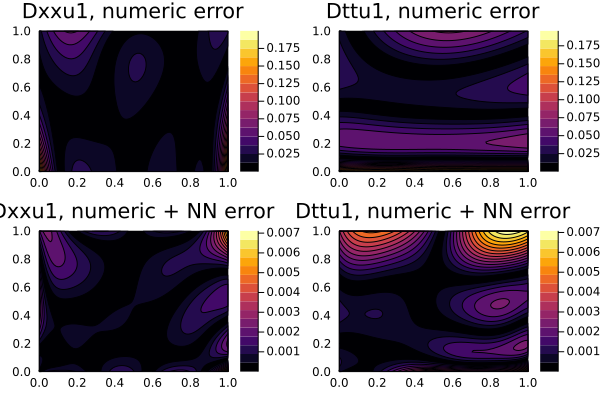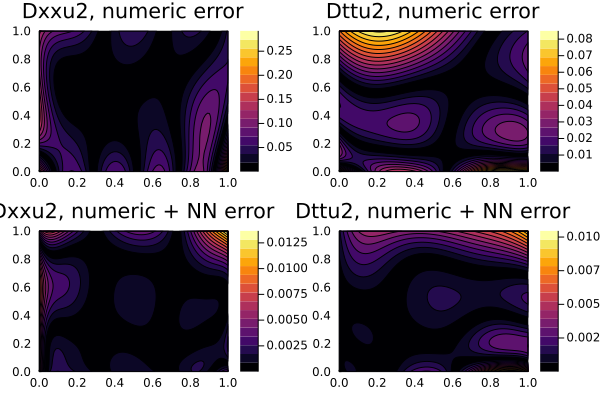## Solving Matrices of PDEs

Also, in addition to systems, we can use the matrix form of PDEs:

@parameters x y
@variables u[1:2,1:2](..)
@derivatives Dxx''~x
@derivatives Dyy''~y

# matrix PDE
eqs  = @. [(Dxx(u_(x,y)) + Dyy(u_(x,y))) for u_ in u] ~ -sin(pi*x)*sin(pi*y)*[0 1; 0 1]

# Initial and boundary conditions
bcs = [u(x,0) ~ x, u(x,0) ~ 2, u(x,0) ~ 3, u(x,0) ~ 4]

## Linear parabolic system of PDEs

We can use NeuralPDE to solve the linear parabolic system of PDEs:

\begin{aligned} \frac{\partial u}{\partial t} &= a * \frac{\partial^2 u}{\partial x^2} + b_1 u + c_1 w \\ \frac{\partial w}{\partial t} &= a * \frac{\partial^2 w}{\partial x^2} + b_2 u + c_2 w \\ \end{aligned}

with initial and boundary conditions:

\begin{aligned} u(0, x) = \frac{b_1 - \lambda_2}{b_2 (\lambda_1 - \lambda_2)} \cdot cos(\frac{x}{a}) - \frac{b_1 - \lambda_1}{b_2 (\lambda_1 - \lambda_2)} \cdot cos(\frac{x}{a}) \\ w(0, x) = 0 \\ u(t, 0) = \frac{b_1 - \lambda_2}{b_2 (\lambda_1 - \lambda_2)} \cdot e^{\lambda_1t} - \frac{b_1 - \lambda_1}{b_2 (\lambda_1 - \lambda_2)} \cdot e^{\lambda_2t} \\ w(t, 0) = \frac{e^{\lambda_1}-e^{\lambda_2}}{\lambda_1 - \lambda_2} \\ u(t, 1) = \frac{b_1 - \lambda_2}{b_2 (\lambda_1 - \lambda_2)} \cdot e^{\lambda_1t} \cdot cos(\frac{x}{a}) - \frac{b_1 - \lambda_1}{b_2 (\lambda_1 - \lambda_2)} \cdot e^{\lambda_2t} * cos(\frac{x}{a}) \\ w(t, 1) = \frac{e^{\lambda_1} cos(\frac{x}{a})-e^{\lambda_2}cos(\frac{x}{a})}{\lambda_1 - \lambda_2} \end{aligned}

with a physics-informed neural network.

using NeuralPDE, Flux, ModelingToolkit, GalacticOptim, Optim, DiffEqFlux
using Plots
import ModelingToolkit: Interval, infimum, supremum

@parameters t, x
@variables u(..), w(..)
Dxx = Differential(x)^2
Dt = Differential(t)

# Constants
a  = 1
b1 = 4
b2 = 2
c1 = 3
c2 = 1
λ1 = (b1 + c2 + sqrt((b1 + c2)^2 + 4 * (b1 * c2 - b2 * c1))) / 2
λ2 = (b1 + c2 - sqrt((b1 + c2)^2 + 4 * (b1 * c2 - b2 * c1))) / 2

# Analytic solution
θ(t, x) = exp(-t) * cos(x / a)
u_analytic(t, x) = (b1 - λ2) / (b2 * (λ1 - λ2)) * exp(λ1 * t) * θ(t, x) - (b1 - λ1) / (b2 * (λ1 - λ2)) * exp(λ2 * t) * θ(t, x)
w_analytic(t, x) = 1 / (λ1 - λ2) * (exp(λ1 * t) * θ(t, x) - exp(λ2 * t) * θ(t, x))

# Second-order constant-coefficient linear parabolic system
eqs = [Dt(u(x, t)) ~ a * Dxx(u(x, t)) + b1 * u(x, t) + c1 * w(x, t),
Dt(w(x, t)) ~ a * Dxx(w(x, t)) + b2 * u(x, t) + c2 * w(x, t)]

# Boundary conditions
bcs = [u(0, x) ~ u_analytic(0, x),
w(0, x) ~ w_analytic(0, x),
u(t, 0) ~ u_analytic(t, 0),
w(t, 0) ~ w_analytic(t, 0),
u(t, 1) ~ u_analytic(t, 1),
w(t, 1) ~ w_analytic(t, 1)]

# Space and time domains
domains = [x ∈ Interval(0.0, 1.0),
t ∈ Interval(0.0, 1.0)]

# Neural network
input_ = length(domains)
n = 15
chain = [FastChain(FastDense(input_, n, Flux.σ), FastDense(n, n, Flux.σ), FastDense(n, 1)) for _ in 1:2]
initθ = map(c -> Float64.(c), DiffEqFlux.initial_params.(chain))

discretization = PhysicsInformedNN(chain, _strategy, init_params=initθ)

@named pde_system = PDESystem(eqs, bcs, domains, [t,x], [u(t,x),w(t,x)])
prob = discretize(pde_system, discretization)
sym_prob = symbolic_discretize(pde_system, discretization)

pde_inner_loss_functions = prob.f.f.loss_function.pde_loss_function.pde_loss_functions.contents
bcs_inner_loss_functions = prob.f.f.loss_function.bcs_loss_function.bc_loss_functions.contents

cb = function (p, l)
println("loss: ", l)
println("pde_losses: ", map(l_ -> l_(p), pde_inner_loss_functions))
println("bcs_losses: ", map(l_ -> l_(p), bcs_inner_loss_functions))
return false
end

res = GalacticOptim.solve(prob, BFGS(); cb=cb, maxiters=5000)

phi = discretization.phi

# Analysis
ts, xs = [infimum(d.domain):0.01:supremum(d.domain) for d in domains]

acum =  [0;accumulate(+, length.(initθ))]
sep = [acum[i] + 1:acum[i + 1] for i in 1:length(acum) - 1]
minimizers_ = [res.minimizer[s] for s in sep]

analytic_sol_func(t,x) = [u_analytic(t, x), w_analytic(t, x)]
u_real  = [[analytic_sol_func(t, x)[i] for t in ts for x in xs] for i in 1:2]
u_predict  = [[phi[i]([t,x], minimizers_[i]) for t in ts  for x in xs] for i in 1:2]
diff_u = [abs.(u_real[i] .- u_predict[i]) for i in 1:2]
for i in 1:2
p1 = plot(ts, xs, u_real[i], linetype=:contourf, title="u$i, analytic"); p2 = plot(ts, xs, u_predict[i], linetype=:contourf, title="predict"); p3 = plot(ts, xs, diff_u[i], linetype=:contourf, title="error"); plot(p1, p2, p3) savefig("sol_u$i")
end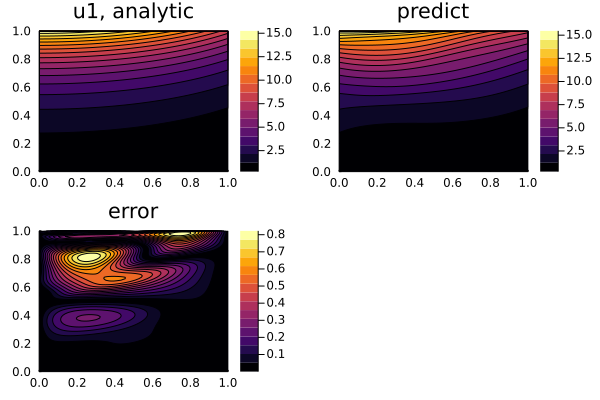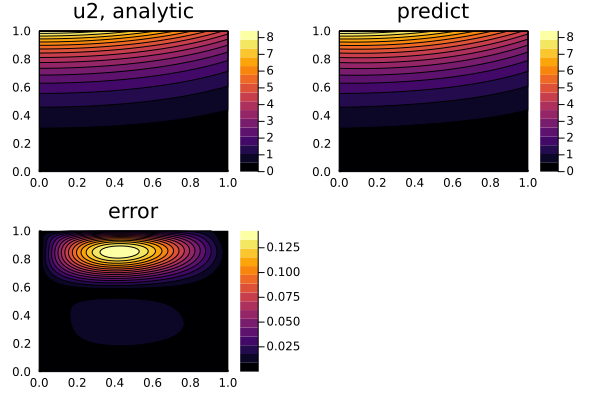## Nonlinear elliptic system of PDEs

We can also solve nonlinear systems such as the system of nonlinear elliptic PDEs

\begin{aligned} \frac{\partial^2u}{\partial x^2} + \frac{\partial^2u}{\partial y^2} = uf(\frac{u}{w}) + \frac{u}{w}h(\frac{u}{w}) \\ \frac{\partial^2w}{\partial x^2} + \frac{\partial^2w}{\partial y^2} = wg(\frac{u}{w}) + h(\frac{u}{w}) \\ \end{aligned}

where f, g, h are arbitrary functions. With initial and boundary conditions:

\begin{aligned} u(0,y) = y + 1 \\ w(1, y) = [cosh(\sqrt[]{f(k)}) + sinh(\sqrt[]{f(k)})]\cdot(y + 1) \\ w(x,0) = cosh(\sqrt[]{f(k)}) + sinh(\sqrt[]{f(k)}) \\ w(0,y) = k(y + 1) \\ u(1, y) = k[cosh(\sqrt[]{f(k)}) + sinh(\sqrt[]{f(k)})]\cdot(y + 1) \\ u(x,0) = k[cosh(\sqrt[]{f(k)}) + sinh(\sqrt[]{f(k)})] \\ \end{aligned}

where k is a root of the algebraic (transcendental) equation f(k) = g(k).

This is done using a derivative neural network approximation.

using NeuralPDE, Flux, ModelingToolkit, GalacticOptim, Optim, DiffEqFlux, DifferentialEquations, Roots
using Plots
import ModelingToolkit: Interval, infimum, supremum

@parameters x, y
Dx = Differential(x)
Dy = Differential(y)
@variables Dxu(..), Dyu(..), Dxw(..), Dyw(..)
@variables u(..), w(..)

# Arbitrary functions
f(x) = sin(x)
g(x) = cos(x)
h(x) = x
root(x) = f(x) - g(x)

# Analytic solution
k = find_zero(root, (0, 1), Bisection())                            # k is a root of the algebraic (transcendental) equation f(x) = g(x)
θ(x, y) = (cosh(sqrt(f(k)) * x) + sinh(sqrt(f(k)) * x)) * (y + 1)   # Analytical solution to Helmholtz equation
w_analytic(x, y) = θ(x, y) - h(k) / f(k)
u_analytic(x, y) = k * w_analytic(x, y)

# Nonlinear Steady-State Systems of Two Reaction-Diffusion Equations with 3 arbitrary function f, g, h
eqs_ = [Dx(Dxu(x, y)) + Dy(Dyu(x, y)) ~ u(x, y) * f(u(x, y) / w(x, y)) + u(x, y) / w(x, y) * h(u(x, y) / w(x, y)),
Dx(Dxw(x, y)) + Dy(Dyw(x, y)) ~ w(x, y) * g(u(x, y) / w(x, y)) + h(u(x, y) / w(x, y))]

# Boundary conditions
bcs_ = [u(0, y) ~ u_analytic(0, y),
u(1, y) ~ u_analytic(1, y),
u(x, 0) ~ u_analytic(x, 0),
w(0, y) ~ w_analytic(0, y),
w(1, y) ~ w_analytic(1, y),
w(x, 0) ~ w_analytic(x, 0)]

der_ = [Dy(u(x, y)) ~ Dyu(x, y),
Dy(w(x, y)) ~ Dyw(x, y),
Dx(u(x, y)) ~ Dxu(x, y),
Dx(w(x, y)) ~ Dxw(x, y)]

bcs__ = [bcs_;der_]

# Space and time domains
domains = [x ∈ Interval(0.0, 1.0),
y ∈ Interval(0.0, 1.0)]

# Neural network
input_ = length(domains)
n = 15
chain = [FastChain(FastDense(input_, n, Flux.σ), FastDense(n, n, Flux.σ), FastDense(n, 1)) for _ in 1:6] # 1:number of @variables
initθ = map(c -> Float64.(c), DiffEqFlux.initial_params.(chain))

discretization = PhysicsInformedNN(chain, _strategy, init_params=initθ)

vars = [u(x,y),w(x,y),Dxu(x,y),Dyu(x,y),Dxw(x,y),Dyw(x,y)]
@named pde_system = PDESystem(eqs_, bcs__, domains, [x,y], vars)
prob = NeuralPDE.discretize(pde_system, discretization)
sym_prob = NeuralPDE.symbolic_discretize(pde_system, discretization)

pde_inner_loss_functions = prob.f.f.loss_function.pde_loss_function.pde_loss_functions.contents
inner_loss_functions = prob.f.f.loss_function.bcs_loss_function.bc_loss_functions.contents
bcs_inner_loss_functions = inner_loss_functions[1:6]
aprox_derivative_loss_functions = inner_loss_functions[7:end]

cb = function (p, l)
println("loss: ", l)
println("pde_losses: ", map(l_ -> l_(p), pde_inner_loss_functions))
println("bcs_losses: ", map(l_ -> l_(p), bcs_inner_loss_functions))
println("der_losses: ", map(l_ -> l_(p), aprox_derivative_loss_functions))
return false
end

res = GalacticOptim.solve(prob, BFGS(); cb=cb, maxiters=5000)

phi = discretization.phi

# Analysis
xs, ys = [infimum(d.domain):0.01:supremum(d.domain) for d in domains]

acum =  [0;accumulate(+, length.(initθ))]
sep = [acum[i] + 1:acum[i + 1] for i in 1:length(acum) - 1]
minimizers_ = [res.minimizer[s] for s in sep]

analytic_sol_func(x,y) = [u_analytic(x, y), w_analytic(x, y)]
u_real  = [[analytic_sol_func(x, y)[i] for x in xs for y in ys] for i in 1:2]
u_predict  = [[phi[i]([x,y], minimizers_[i]) for x in xs for y in ys] for i in 1:2]
diff_u = [abs.(u_real[i] .- u_predict[i]) for i in 1:2]
for i in 1:2
p1 = plot(xs, ys, u_real[i], linetype=:contourf, title="u$i, analytic"); p2 = plot(xs, ys, u_predict[i], linetype=:contourf, title="predict"); p3 = plot(xs, ys, diff_u[i], linetype=:contourf, title="error"); plot(p1, p2, p3) savefig("non_linear_elliptic_sol_u$i")
end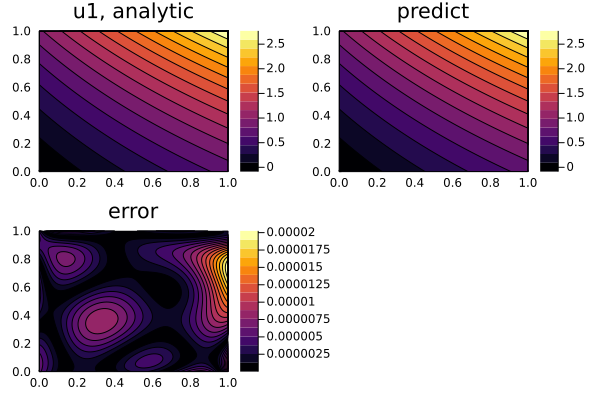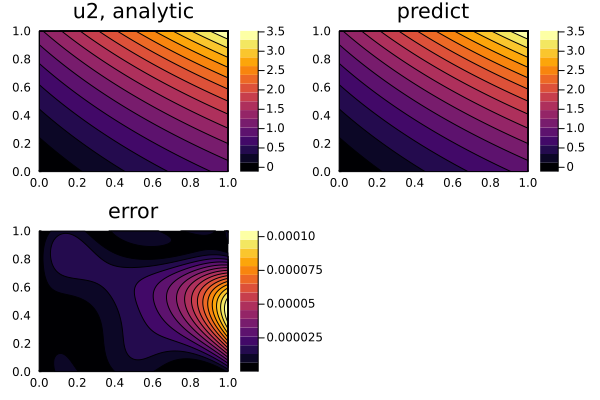## Nonlinear hyperbolic system of PDEs

Lastly, we may also solve hyperbolic systems like the following

\begin{aligned} \frac{\partial^2u}{\partial t^2} = \frac{a}{x^n} \frac{\partial}{\partial x}(x^n \frac{\partial u}{\partial x}) + u f(\frac{u}{w}) \\ \frac{\partial^2w}{\partial t^2} = \frac{b}{x^n} \frac{\partial}{\partial x}(x^n \frac{\partial u}{\partial x}) + w g(\frac{u}{w}) \\ \end{aligned}

where f and g are arbitrary functions. With initial and boundary conditions:

\begin{aligned} u(0,x) = k * [j0(ξ(0, x)) + y0(ξ(0, x))] \\ u(t,0) = k * [j0(ξ(t, 0)) + y0(ξ(t, 0))] \\ u(t,1) = k * [j0(ξ(t, 1)) + y0(ξ(t, 1))] \\ w(0,x) = j0(ξ(0, x)) + y0(ξ(0, x)) \\ w(t,0) = j0(ξ(t, 0)) + y0(ξ(t, 0)) \\ w(t,1) = j0(ξ(t, 0)) + y0(ξ(t, 0)) \\ \end{aligned}

where k is a root of the algebraic (transcendental) equation f(k) = g(k), j0 and y0 are the Bessel functions, and ξ(t, x) is:

\begin{aligned} \frac{\sqrt[]{f(k)}}{\sqrt[]{\frac{a}{x^n}}}\sqrt[]{\frac{a}{x^n}(t+1)^2 - (x+1)^2} \end{aligned}

We solve this with Neural:

using NeuralPDE, Flux, ModelingToolkit, GalacticOptim, Optim, DiffEqFlux, Roots
using SpecialFunctions
using Plots
import ModelingToolkit: Interval, infimum, supremum

@parameters t, x
@variables u(..), w(..)
Dx = Differential(x)
Dt = Differential(t)
Dtt = Differential(t)^2

# Constants
a = 16
b = 16
n = 0

# Arbitrary functions
f(x) = x^2
g(x) = 4 * cos(π * x)
root(x) = g(x) - f(x)

# Analytic solution
k = find_zero(root, (0, 1), Bisection())                # k is a root of the algebraic (transcendental) equation f(x) = g(x)
ξ(t, x) = sqrt(f(k)) / sqrt(a) * sqrt(a * (t + 1)^2 - (x + 1)^2)
θ(t, x) = besselj0(ξ(t, x)) + bessely0(ξ(t, x))                     # Analytical solution to Klein-Gordon equation
w_analytic(t, x) = θ(t, x)
u_analytic(t, x) = k * θ(t, x)

# Nonlinear system of hyperbolic equations
eqs = [Dtt(u(t, x)) ~ a / (x^n) * Dx(x^n * Dx(u(t, x))) + u(t, x) * f(u(t, x) / w(t, x)),
Dtt(w(t, x)) ~ b / (x^n) * Dx(x^n * Dx(w(t, x))) + w(t, x) * g(u(t, x) / w(t, x))]

# Boundary conditions
bcs = [u(0, x) ~ u_analytic(0, x),
w(0, x) ~ w_analytic(0, x),
u(t, 0) ~ u_analytic(t, 0),
w(t, 0) ~ w_analytic(t, 0),
u(t, 1) ~ u_analytic(t, 1),
w(t, 1) ~ w_analytic(t, 1)]

# Space and time domains
domains = [t ∈ Interval(0.0, 1.0),
x ∈ Interval(0.0, 1.0)]

# Neural network
input_ = length(domains)
n = 15
chain = [FastChain(FastDense(input_, n, Flux.σ), FastDense(n, n, Flux.σ), FastDense(n, 1)) for _ in 1:2]
initθ = map(c -> Float64.(c), DiffEqFlux.initial_params.(chain))

discretization = PhysicsInformedNN(chain, _strategy, init_params=initθ)

@named pde_system = PDESystem(eqs, bcs, domains, [t,x], [u(t,x),w(t,x)])
prob = discretize(pde_system, discretization)
sym_prob = symbolic_discretize(pde_system, discretization)

pde_inner_loss_functions = prob.f.f.loss_function.pde_loss_function.pde_loss_functions.contents
bcs_inner_loss_functions = prob.f.f.loss_function.bcs_loss_function.bc_loss_functions.contents

cb = function (p, l)
println("loss: ", l)
println("pde_losses: ", map(l_ -> l_(p), pde_inner_loss_functions))
println("bcs_losses: ", map(l_ -> l_(p), bcs_inner_loss_functions))
return false
end

res = GalacticOptim.solve(prob, BFGS(); cb=cb, maxiters=1000)

phi = discretization.phi

# Analysis
ts, xs = [infimum(d.domain):0.01:supremum(d.domain) for d in domains]

acum =  [0;accumulate(+, length.(initθ))]
sep = [acum[i] + 1:acum[i + 1] for i in 1:length(acum) - 1]
minimizers_ = [res.minimizer[s] for s in sep]

analytic_sol_func(t,x) = [u_analytic(t, x), w_analytic(t, x)]
u_real  = [[analytic_sol_func(t, x)[i] for t in ts for x in xs] for i in 1:2]
u_predict  = [[phi[i]([t,x], minimizers_[i]) for t in ts  for x in xs] for i in 1:2]
diff_u = [abs.(u_real[i] .- u_predict[i]) for i in 1:2]
for i in 1:2
p1 = plot(ts, xs, u_real[i], linetype=:contourf, title="u$i, analytic"); p2 = plot(ts, xs, u_predict[i], linetype=:contourf, title="predict"); p3 = plot(ts, xs, diff_u[i], linetype=:contourf, title="error"); plot(p1, p2, p3) savefig("nonlinear_hyperbolic_sol_u$i")
end